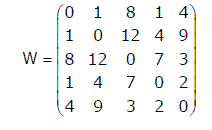# Data Structures and Algorithms | Set 25

• Difficulty Level : Hard
• Last Updated : 11 Nov, 2021

Following questions have been asked in GATE 2010 exam.

1 Consider a complete undirected graph with vertex set {0, 1, 2, 3, 4}. Entry Wij in the matrix W below is the weight of the edge {i, j}.What is the minimum possible weight of a spanning tree T in this graph such that vertex 0 is a leaf node in the tree T?

(A) 7
(B) 8
(C) 9
(D) 10

To get the minimum spanning tree with vertex 0 as leaf, first remove 0th row and 0th column and then get the minimum spanning tree (MST) of the remaining graph. Once we have MST of the remaining graph, connect the MST to vertex 0 with the edge with minimum weight (we have two options as there are two 1s in 0th row).

2. In the graph given in question 1, what is the minimum possible weight of a path P from vertex 1 to vertex 2 in this graph such that P contains at most 3 edges?

(A) 7
(B) 8
(C) 9
(D) 10

Path: 1 -> 0 -> 4 -> 2
Weight: 1 + 4 + 3

3. The degree sequence of a simple graph is the sequence of the degrees of the nodes in the graph in decreasing order. Which of the following sequences can not be the degree sequence of any graph?
I. 7, 6, 5, 4, 4, 3, 2, 1
II. 6, 6, 6, 6, 3, 3, 2, 2
III. 7, 6, 6, 4, 4, 3, 2, 2
IV. 8, 7, 7, 6, 4, 2, 1, 1

(A) I and II
(B) III and IV
(C) IV only
(D) II and IV

In sequence IV, we have a vertex with degree 8 which is not possible in a simple graph (no self loops and no multiple edges) with total vertex count as 8. Maximum possible degree in such a graph is 7.

In sequence II, four vertices are connected to 6 other vertices, but remaining 4 vertices have degrees as 3, 3, 2 and 2 which are not possible in a simple graph (no self loops and no multiple edges).

4. Consider a B+-tree in which the maximum number of keys in a node is 5. What is the minimum number of keys in any non-root node?
(A) 1
(B) 2
(C) 3
(D) 4